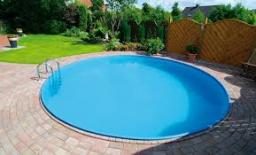# Calculate 3167

The area of the two circles is in a 4:9 ratio. The larger circle has a diameter of 12 cm. Calculate the radius of the smaller circle.

r1 =  4 cm

### Step-by-step explanation:Did you find an error or inaccuracy? Feel free to write us. Thank you!

Tips for related online calculators
Check out our ratio calculator.

#### Grade of the word problem:

We encourage you to watch this tutorial video on this math problem: